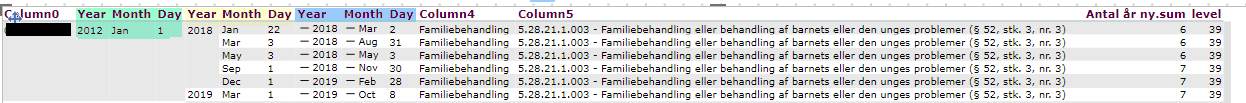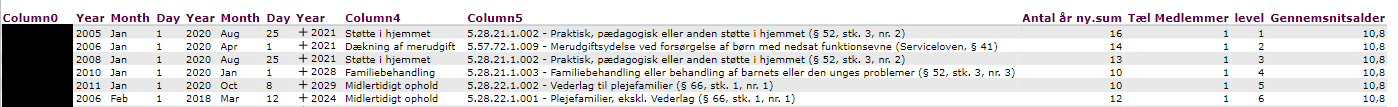# Hide all but 1st child

Hello!

I am currently trying to figure out if there is a way to hide all but the first "child" in a crosstab. Is there a way I can do that with visual agents?

For example if we have:

*Column* T-Shirt | *Column* Date Sales

Red T-Shirt          | 7/27/21 6:02am

| 7/27/21 7:38am

| 7/27/21 9:45am

I would only want to show the first child "7/27/21 6:02am".

Any tips or suggestions are appreciated!

• Hi Tyler

This should do the trick, you probably need to tweak it to fit your data though.

Create a new column, I named it filter

`if sum(d1, 0, m1) = sum(d1, 0(l1), m1) then 1 else if count(d1, d1:0(s), m1) = 1 then 1 else 0`

The

`if sum(d1, 0, m1) = sum(d1, 0(l1), m1) then 1`

Is to ensure that we always show the the parrent.

and

`else if count(d1, d1:0(s), m1) = 1 then 1 else 0`

Counts which row a child is, if it is the 1'st we want 1 as result, otherwise 0.

Finally you use a visibility agent to hide where the filter = 0

• Hello!

Thank you for your answer but it seems targit is not liking the "sum(d1, 0(l1), m1)", I keep getting undefined for the first step.

• Sounds like you data dimension is not a hierarchy but two dimensions instead?

If so this should work

`if sum(d1, 0, m1) = sum(d1, 0(l(1,0)), m1) then 1 else if count(d1, d1:0(s), m1) = 1 then 1 else 0`
• Kristoffer Drejer

That worked!! Thank you so much!!!

• I've tried your suggestion in one of my analyzes, but I can not make it work.

I have 6 dimensions (column0 (person ID), green date, yellow date, blue date, column4 and column5) and would like to hide  all but the first child.. Just as TylerIn the column 'Level' i used

sum(d1, 0(l(1,0,0,0,0,0)), m1)

and it seems like it can sum the years, but when i replace sum with count it say 1 all the way down

Do you have an idea how i solve my problem?

• Hi Gia

Hands down that issue is above what I can see a solution for.

Tyler's case was easy as he only had one child.

But in you case you have 5 Parrent / Childs, so that is a lot of if statements, which I can't wrap my head around.

• Hi

I ended up solving the problem myself.

First, I made af column 'Level' and used

allcount(d1, d1:0(l(1,0,0,0,0,0)), m1)

This gave 'child' to my level 1 (person ID) the same number.

Next, I made an other column 'Tæl medlemmer' where I used

if sum(c2, 0, m1, 0) = sum(c2, -1, m1, 0) then 0 else 1

This gave each of the 'first children' 1 and the others 0, which meant I could hide all the '0 - children' with a  'Visibility agent'